# Convert Booleans to Integers and vice versa in PythonLast updated: Feb 20, 2023
7 min## #Convert Booleans to Integers in Python

Use the `int()` class to convert boolean values to integers, e.g. `result = int(True)`.

The `int()` class will return 1 for `True` boolean values and 0 for `False` values.

main.py
```Copied!```# ✅ convert True and False to 1 and 0

bool_t = True
int_1 = int(bool_t)
print(int_1)  # 👉️ 1

bool_f = False
int_0 = int(bool_f)
print(int_0)  # 👉️ 0

# -----------------------------------------------

# ✅ convert 'true' and 'false' to 1 and 0

str_t = 'true'
int_1 = int(str_t.lower() == 'true')
print(int_1)  # 👉️ 1

str_f = 'false'
int_0 = int(str_f.lower() == 'true')
print(int_0)  # 👉️ 0
``````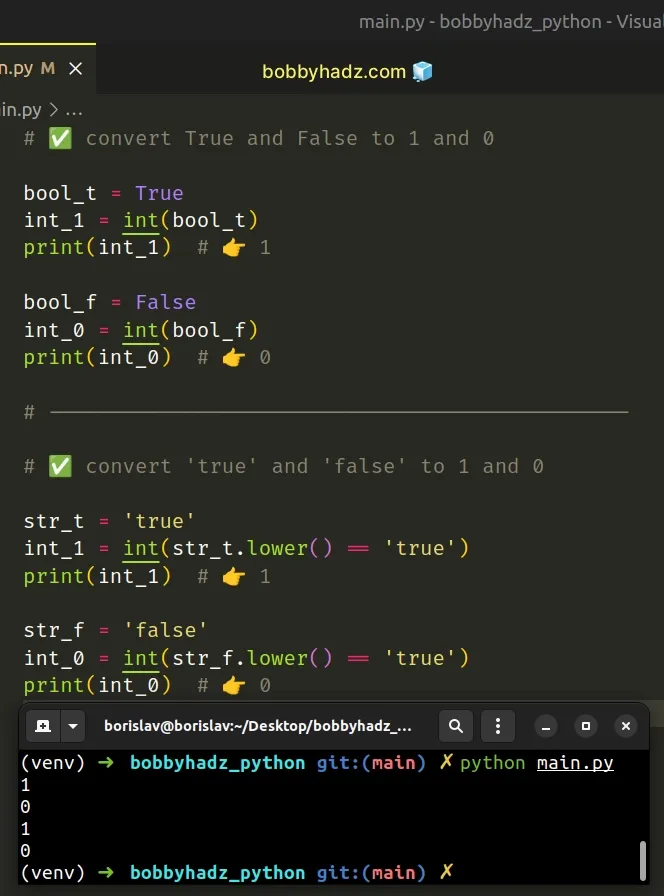If you need to convert integer values to booleans, click on the following subheading:

We used the `int()` class to convert `True` to 1 and `False` to 0.

main.py
```Copied!```result_1 = int(True)
print(result_1) # 👉️ 1

result_2 = int(False)
print(result_2) # 👉️ 0
``````

The int class returns an integer object constructed from the provided argument.

The constructor returns `0` if no arguments are given.

`True` boolean values return `1` after conversion to integers and `False` values return `0`.

## #Convert a list of Booleans to a List of Integers in Python

To convert a list of booleans to a list of integers:

1. Use a list comprehension to iterate over the list.
2. Use the `int()` class to convert each boolean to an integer.
3. The new list will only contain integers.
main.py
```Copied!```list_of_booleans = [True, False, False, True]

list_of_integers = [int(item) for item in list_of_booleans]

print(list_of_integers)  # 👉️ [1, 0, 0, 1]
``````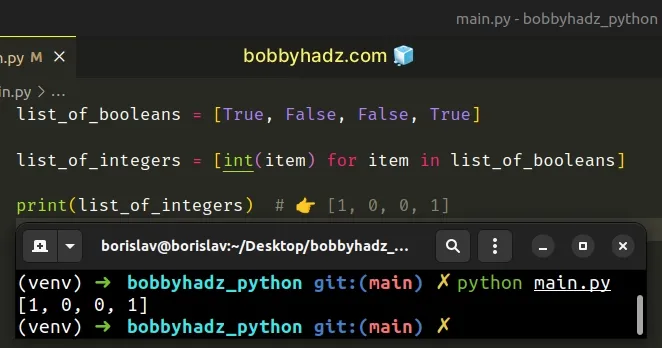We used a list comprehension to convert a list of booleans to a list of integers.

List comprehensions are used to perform some operation for every element, or select a subset of elements that meet a condition.

On each iteration, we pass the current boolean to the `int()` class to convert it to an integer.

main.py
```Copied!```list_of_booleans = [True, False, False, True]

list_of_integers = [int(item) for item in list_of_booleans]

print(list_of_integers)  # 👉️ [1, 0, 0, 1]
``````

The new list contains the integer representation of the booleans in the original list.

## #Convert 'true' and 'false' to 1 and 0 in Python

To convert 'true' values to 1 and 'false' to 0:

1. Use the equality operator to check if the value is equal to the string 'true'.
2. Convert the result of the comparison to an integer.
3. The conversion will return `1` for 'true' values and `0` for 'false' values.
main.py
```Copied!```str_t = 'true'
int_1 = int(str_t.lower() == 'true')
print(int_1)  # 👉️ 1

# ----------------------------------------

str_f = 'false'
int_0 = int(str_f.lower() == 'true')
print(int_0)  # 👉️ 0
``````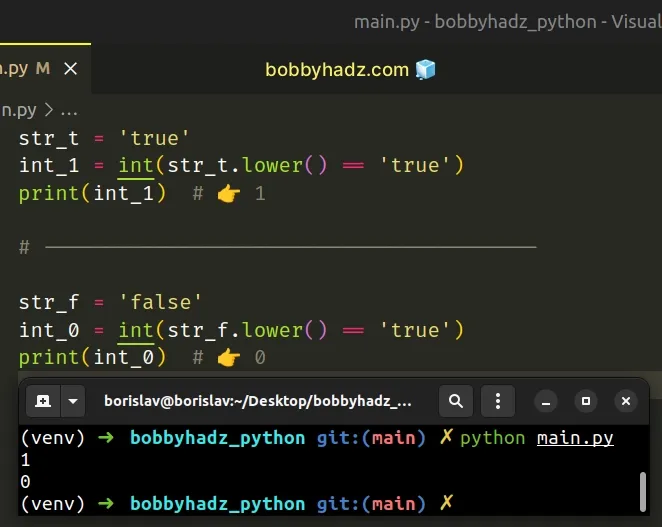We used the `lower()` method to convert the string to lowercase before comparing it to the string `true`.

The str.lower method returns a copy of the string with all the cased characters converted to lowercase.

The method doesn't change the original string, it returns a new string. Strings are immutable in Python.

The equality comparison will return `True` if the string stores a 'true' value and `False` otherwise.

Converting `True` to an integer returns `1` and converting `False` to an integer returns `0`.

## #Convert a list of 'true' and 'false' values to integers

If you need to convert a list of 'true' and 'false' strings to a list of `1` and `0`, use a list comprehension.

main.py
```Copied!```my_list = ['true', 'true', 'false']

result = [int(item.lower() == 'true') for item in my_list]

print(result)  # 👉️ [1, 1, 0]
``````

On each iteration, we compare the current string to the string 'true' and convert the result of the comparison to an integer.

Strings storing a 'true' value get converted to `1` and all other strings get converted to `0`.

## #Convert a List of Booleans to List of Integers using map()

This is a three-step process:

1. Pass the `int()` class and the list to the `map()` function.
2. The `map()` function will call the `int()` class with each boolean in the list.
3. Use the `list()` class to convert the `map` object to a list.
main.py
```Copied!```list_of_booleans = [True, False, False, True]

list_of_integers = list(map(int, list_of_booleans))

print(list_of_integers)  # 👉️ [1, 0, 0, 1]
``````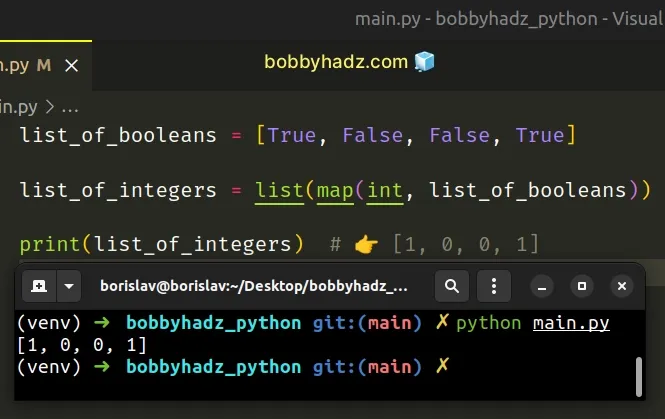The `map()` function takes a function and an iterable as arguments and calls the function with each item of the iterable.

The `int()` class gets passed each boolean in the list and converts each value to an integer.

Lastly, we used the `list()` class to convert the map object to a list.

The `list` class takes an iterable and returns a list object.

## #Convert an Array of Booleans to an Array of Integers using NumPy

Use the `astype()` method to convert an array of booleans to an array of integers.

main.py
```Copied!```import numpy as np

arr = np.array([True, False, False, True], dtype=bool)

int_arr = arr.astype(int)
print(int_arr)  # 👉️ [1 0 0 1]
``````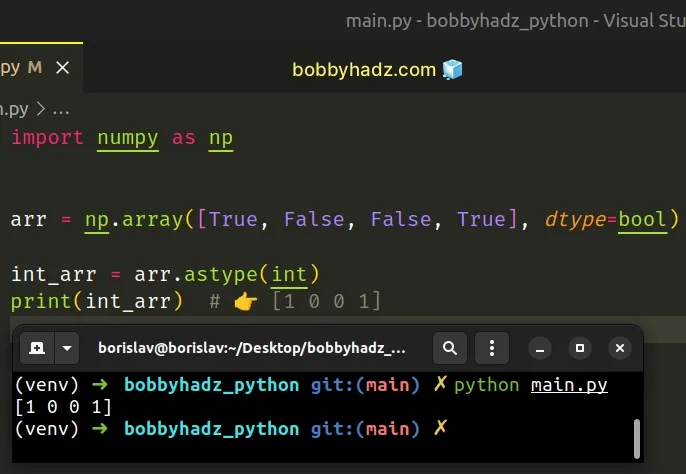The `astype()` method will return a copy of the array that contains the integer representation of the values.

Make sure you have NumPy installed to be able to run the code sample.

shell
```Copied!```pip install numpy

pip3 install numpy
``````

You can use the astype method on a NumPy array to copy the array and cast it to a specified type.

The only parameter we passed to the `astype` method is the data type to which the array is cast.

To convert the array of booleans to an array of integers, pass the `int` class to the `astype()` method.

## #Convert Integers to Booleans in Python

Use the `bool()` class to convert the numbers 1 and 0 to boolean values.

The `bool()` class will return `True` when converting 1 to a boolean and `False` when converting 0 to a boolean.

main.py
```Copied!```# ✅ convert 1 and 0 to boolean values
result = bool(1)
print(result)  # 👉️ True

result = bool(0)
print(result)  # 👉️ False

# ------------------------------------------

# ✅ convert boolean values to integers
result = int(True)
print(result)  # 👉️ 1

result = int(False)
print(result)  # 👉️ 0
``````

We used the `bool()` class to convert the numbers 1 and 0 to booleans.

main.py
```Copied!```result = bool(1)
print(result)  # 👉️ True

result = bool(0)
print(result)  # 👉️ False
``````

The bool() class takes a value and converts it to a boolean (True or False). If the provided value is falsy or omitted, then `bool` returns `False`, otherwise it returns `True`.

All values that are not truthy are considered falsy. The falsy values in Python are:

• constants defined to be falsy: `None` and `False`.
• `0` (zero) of any numeric type.
• empty sequences and collections: `""` (empty string), `()` (empty tuple), `[]` (empty list), `{}` (empty dictionary), `set()` (empty set), `range(0)` (empty range).

The `bool()` class returns `True` if passed any non-falsy value.

In other words, the `bool()` class will return `True` when called with any number other than `0`.

If you need to convert a boolean value to an integer, use the `int()` class.

main.py
```Copied!```result = int(True)
print(result)  # 👉️ 1

result = int(False)
print(result)  # 👉️ 0
``````

The `int` class returns an integer object constructed from the provided number or string argument.

The constructor returns `0` if no arguments are given.

## #Convert a list of Integers to a list of Booleans

To convert a list of integers to a list of booleans:

1. Use a list comprehension to iterate over the list.
2. Use the `bool()` class to convert each integer to a boolean.
3. The new list will only contain boolean values.
main.py
```Copied!```list_of_integers = [1, 0, 1, 0, 1, 1]

list_of_booleans = [bool(item) for item in list_of_integers]

# 👇️ [True, False, True, False, True, True]
print(list_of_booleans)
``````

We used a list comprehension to iterate over the list of integers.

List comprehensions are used to perform some operation for every element, or select a subset of elements that meet a condition.

On each iteration, we pass the current integer to the `bool()` class to convert it to a boolean.

The new list contains the boolean representation of the integers in the original list.

The `bool()` class takes a value and converts it to a boolean (True or False). If the provided value is falsy or omitted, then `bool` returns `False`, otherwise it returns `True`.

All values that are not truthy are considered falsy. The falsy values in Python are:

• constants defined to be falsy: `None` and `False`.
• `0` (zero) of any numeric type.
• empty sequences and collections: `""` (empty string), `()` (empty tuple), `[]` (empty list), `{}` (empty dictionary), `set()` (empty set), `range(0)` (empty range).

The `bool()` class returns `True` if passed any non-falsy value.

In other words, passing any integer other than 0 to the `bool()` class returns `True`.

Alternatively, you can use the `map()` function.

## #Convert list of integers to list of booleans using map()

This is a three-step process:

1. Pass the `bool()` class and the list to the `map()` function.
2. The `map()` function will call the `bool()` class with each integer in the list.
3. Use the `list()` class to convert the `map` object to a list.
main.py
```Copied!```list_of_integers = [1, 0, 1, 0, 1, 1]

list_of_booleans = list(map(bool, list_of_integers))

# # 👇️ [True, False, True, False, True, True]
print(list_of_booleans)
``````

The map() function takes a function and an iterable as arguments and calls the function with each item of the iterable.

The `bool()` class gets passed each integer in the list and converts each value to a boolean.

Lastly, we used the `list()` class to convert the `map` object to a list.

The list class takes an iterable and returns a list object.